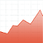V-Lab

## Documentation>Volatility Analysis

Consider a return time series ${r}_{t}$, with $t=1,2,3...T$. The sample variance of these returns is defined as

$σ2⁡rt=1T−1∑t=1T(rt-r¯)2$

where

$r¯=1T∑t=1Trt$

is the sample average of the returns.

This descriptive statistic, the sample variance, is computed using the whole sample, $t=1,2,3...T$. If we compute the variance using only the first half part of the sample and compare it to the variance computed with the second half of the sample, they will likely be different. That is, the variance, or its square root, the volatility, is probably time-varying.

In a intuitive way, the volatility is a measure of how much the return fluctuates around its mean. It is a measure of the risk. Hence, it is useful to have means to compute this volatility at any instant, to analyze how it varies over time and, possibly, to forecast its future values.

More formally, let ${r}_{t}=\mu +{\epsilon }_{t}$ be a return time series, where $\mu$ is the expected return and${\epsilon }_{t}$ is a zero-mean white noise. Although it is serially uncorrelated, the series${\epsilon }_{t}$ does not need to be serially independent. For instance, it can exhibit time varying variances which we describe as conditional heteroskedasticity. That is, its future volatility can be predicted based upon its past volatility and other conditioning variables.

In order to perform volatility analysis, we need to specify this dependence. The Generalized Autoregressive Conditional Heteroskedasticity$\left(\mathrm{GARCH}\right)$ model is an example of such specification.

## Stylized Facts

Some phenomena are systematically observed in almost all return time series. A good conditional heteroskedasticity model should be able to capture most of these empirical facts. In this section we list the most well known stylized facts in volatility analysis.

## Volatility Clusters

The volatility is more likely to be high at time $t$ if it was also high at time $t-1$. That is, a shock at time $t-1$ increases not only the variance at time $t-1$ but also the variance at time $t$. In other words, the markets are more volatile in some periods, and they are more tranquil in others. The volatilities are clustered in time. The $\mathrm{GARCH}$ type models capture this effect very well. In fact, these models are precisely a way to specify how volatility at time $t$ depends on past volatility (and possibly other conditioning variables).

## Fat Tails

Return time series generally present fat tails, also known as excess kurtosis, or leptokurtosis. That is, their kurtosis (the fourth central moment normalized by the square of the variance) is usually greater than three, the kurtosis of a Gaussian random variable. In fact, a popular statistical test for the hypothesis of Gaussianity of a distribution, the Jarque-Bera Test, jointly test both if the distribution is symmetric and if the distribution presents kurtosis equal to three.

If returns are fat-tailed, the probability of having extreme events (very high or very low returns) is higher than it would be in the normal (Gaussian) case.

Most volatility models such as the GARCH model give rise to fat tailed return distributions. This is true whether the underlying shocks are Gaussian or are themselves fat tailed. For estimation it is typically assumed that these underlying shocks are Gaussian, however the estimates are well behaved for large samples even if this is not the case. The estimators are Quasi-Maximum Likelihood or QML estimators in this case and can be shown to be consistent under relatively mild conditions.

## Asymmetry

There is a stylized fact that the plain $\mathrm{GARCH}$ model is not able to capture, which is the empirically observed fact that negative shocks at time $t-1$ have a stronger impact on the variance at time $t$ than positive shocks. However, the $\mathrm{GARCH}$ model can be easily augmented to capture this asymmetry. Examples of these generalizations are the Threshold $\mathrm{GARCH}$ ($\mathrm{TGARCH}$), the Asymmetric $\mathrm{GARCH}$ ($\mathrm{AGARCH}$) and the Exponential $\mathrm{GARCH}$ $\left(\mathrm{EGARCH}\right)$.

This asymmetry used to be called leverage the effect because the increase in risk was believed to come from the increased leverage induced by a negative shock, but now it is recognized that this channel does not explain all the facts and risk aversion is an important mechanism.

## References

Bollerslev, Tim, 1986, “Generalized Autoregressive Conditional Heteroskedasticity”, Journal of Econometrics

Engle, R. F. 1982, “Autoregressive Conditional Heteroskedasticity with Estimates of the Variance of UK Inflation”, Econometrica, pp987-1008

Engle, R. F. and Andrew Patton, 2001, “What Good is a Volatility Model?,”, Quantiative Finance V1N2, pp237-245

Engle, R. F., 2009. Anticipating Correlations: A New Paradigm for Risk Management. Princeton University Press.

Tsay, R. S., 2005. Analysis of Financial Time Series — 2nd Ed. Wiley-Interscience.